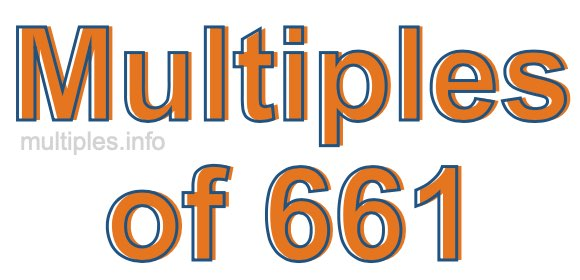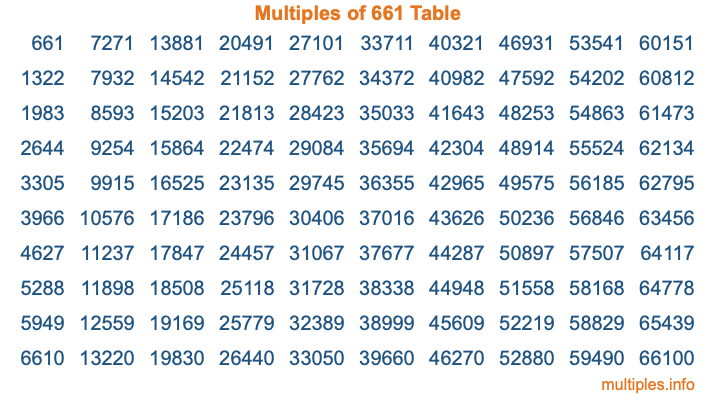Multiples of 661Welcome to the Multiples of 661 page. Here we will first teach you everything you will ever need to know about the multiples of 661, and then give you a study guide summary of everything we taught you to make sure you remember it all. Use this page to look up facts and learn information about the multiples of 661. This page will make you a multiples of six hundred sixty-one expert!

Definition of Multiples of 661
Multiples of 661 are all the numbers that when divided by 661 equal an integer. Each of the multiples of 661 are called a multiple. A multiple of 661 is created by multiplying 661 by an integer.

Therefore, to create a list of multiples of 661, you start with 1 multiplied by 661, then 2 multiplied by 661, then 3 multiplied by 661, and so on for as long as you want. Thus, the list of the first five multiples of 661 is 661, 1322, 1983, 2644, and 3305. To see a larger list of multiples of 661, see the printable image of Multiples of 661 further down on this page. We also have a category where you can choose any nth multiple of 661.

Multiples of 661 Checker
The Multiples of 661 Checker below checks to see if any number of your choice is a multiple of 661. In other words, it checks to see if there is any number (integer) that when multiplied by 661 will equal your number. To do that, we divide your number by 661. If the the quotient is an integer, then your number is a multiple of 661.

Is  a multiple of 661?

Least Common Multiple of 661 and ...
A Least Common Multiple (LCM) is the lowest multiple that two or more numbers have in common. This is also called the smallest common multiple or lowest common multiple and is useful to know when you are adding our subtracting fractions. Enter one or more numbers below (661 is already entered) to find the LCM.

Check out our LCM Calculator if you need more details about the Least Common Multiple or if you need the LCM for different numbers for adding and subtraction fractions.

nth Multiple of 661
As we stated above, 661 is the first multiple of 661, 1322 is the second multiple of 661, 1983 is the third multiple of 661, and so on. Enter a number below to find the nth multiple of 661.

th multiple of 661

Multiples of 661 vs Factors of 661
661 is a multiple of 661 and a factor of 661, but that is where the similarities end. All postive multiples of 661 are 661 or greater than 661. All positive factors of 661 are 661 or less than 661.

Below is the beginning list of multiples of 661 and the factors of 661 so you can compare:

Multiples of 661: 661, 1322, 1983, 2644, 3305, etc.

Factors of 661: 1, 661

As you can see, the multiples of 661 are all the numbers that you can divide by 661 to get a whole number. The factors of 661, on the other hand, are all the whole numbers that you can multiply by another whole number to get 661.

It's also interesting to note that if a number (x) is a factor of 661, then 661 will also be a multiple of that number (x).

Multiples of 661 vs Divisors of 661
The divisors of 661 are all the integers that 661 can be divided by evenly. Below is a list of the divisors of 661.

Divisors of 661: 1, 661

The interesting thing to note here is that if you take any multiple of 661 and divide it by a divisor of 661, you will see that the quotient is an integer.

Multiples of 661 Table
Below is an image of the first 100 multiples of 661 in a table. The table is in chronological order, column by column. The first column has the first ten multiples of 661, the second column has the next ten multiples of 661, and so on.The Multiples of 661 Table is also referred to as the 661 Times Table or Times Table of 661. You are welcome to print out our table for your studies.

Negative Multiples of 661
Although not often discussed or needed in math, it is worth mentioning that you can make a list of negative multiples of 661 by multiplying 661 by -1, then by -2, then by -3, and so on, to get the following list of negative multiples of 661:

-661, -1322, -1983, -2644, -3305, etc.

Multiples of 661 Summary
Below is a summary of important Multiples of 661 facts that we have discussed on this page. To retain the knowledge on this page, we recommend that you read through the summary and explain to yourself or a study partner why they hold true.

There are an infinite number of multiples of 661.

A multiple of 661 divided by 661 will equal a whole number.

661 divided by a factor of 661 equals a divisor of 661.

The nth multiple of 661 is n times 661.

The largest factor of 661 is equal to the first positive multiple of 661.

661 is a multiple of every factor of 661.

661 is a multiple of 661.

A multiple of 661 divided by a divisor of 661 equals an integer.

661 divided by a divisor of 661 equals a factor of 661.

Any integer times 661 will equal a multiple of 661.

Multiples of a Number
Here you can get the multiples of another number, all with the same attention to detail as we did for multiples of 661 on this page.

Multiples of
Multiples of 662
Did you find our page about multiples of six hundred sixty-one educational? Do you want more knowledge? Check out the multiples of the next number on our list!